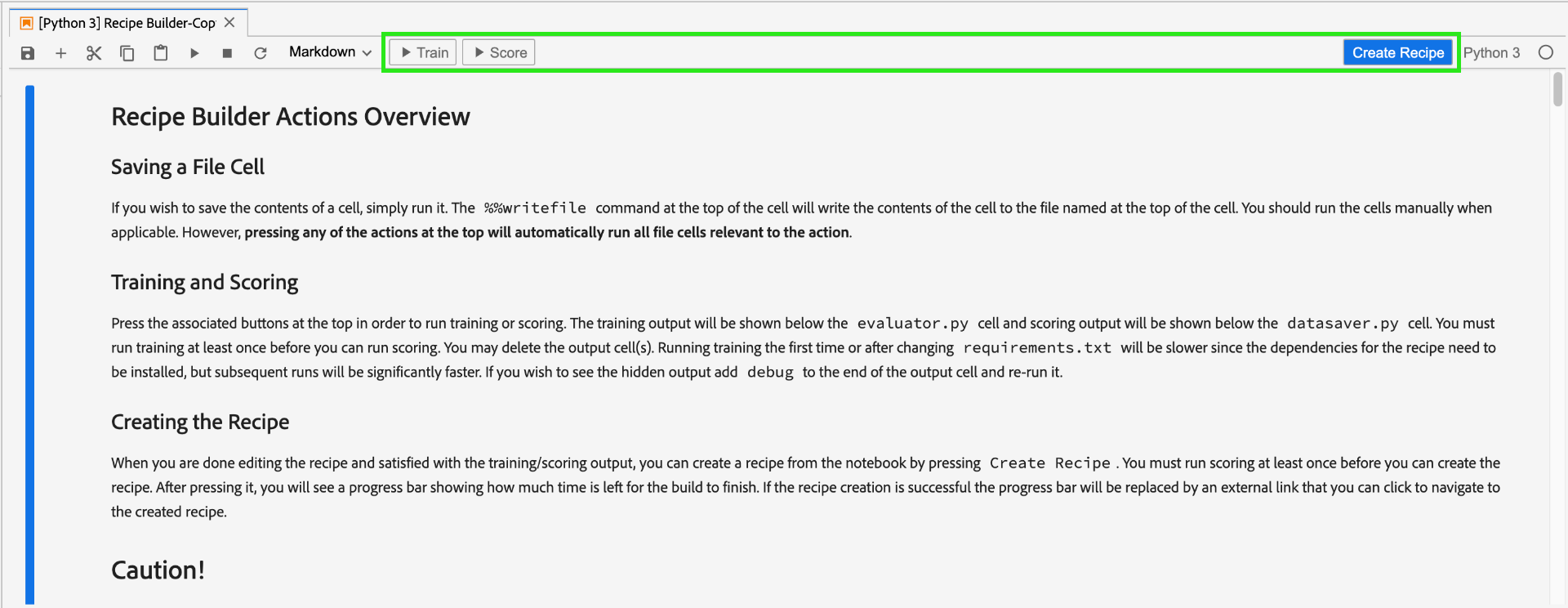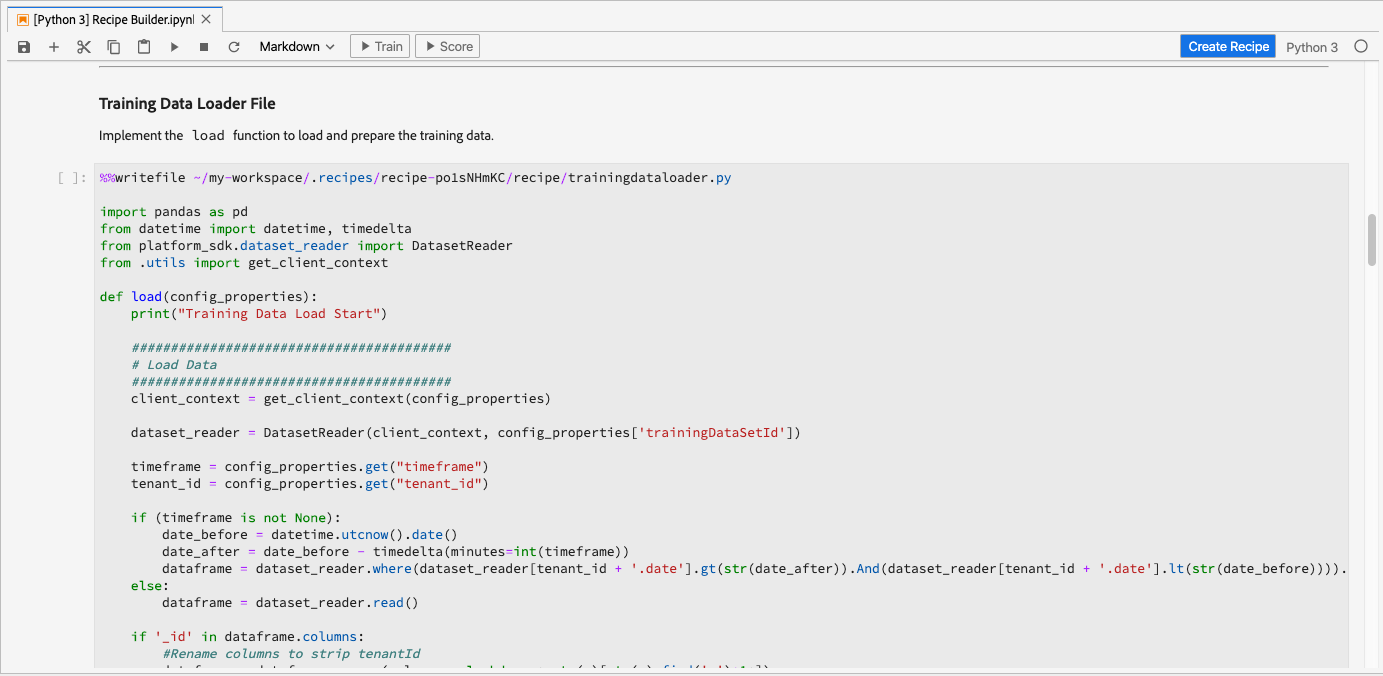# 使用Jupyter Notebooks建立配方

## 引入的概念：

• 模型： 模型是機器學習方式的例項，使用歷史資料和組態進行訓練，以解決商業使用案例。
• 訓練： 訓練是學習模式和從標籤資料獲得見解的過程。
• 計分： 計分是使用訓練好的模型從資料產生見解的程式。

## 開始使用JupyterLab筆記本環境

Recipe Builder筆記型電腦允許您在筆記本內運行培訓和計分。 這可讓您在訓練和計分資料上執行實驗之間，靈活地變更其`train()``score()`方法。 一旦您對培訓和計分的輸出感到滿意，您就可以使用筆記型電腦建立用於Data Science Workspace的配方，以便將配方功能內建在Recipe Builder筆記型電腦上。

Recipe Builder筆記型電腦支援使用所有檔案格式，但目前「建立方式」功能僅支援Python。## 開始使用Recipe Builder筆記型電腦

### 需求檔案

``````python=3.6.7
scikit-learn
pandas
numpy
data_access_sdk_python
``````

### 配置檔案

• `trainingDataSetId`
• `ACP_DSW_TRAINING_XDM_SCHEMA`
• `scoringDataSetId`
• `ACP_DSW_SCORING_RESULTS_XDM_SCHEMA`
• `scoringResultsDataSetId`• `ML_FRAMEWORK_IMS_USER_CLIENT_ID`
• `ML_FRAMEWORK_IMS_TOKEN`
• `ML_FRAMEWORK_IMS_ML_TOKEN`
• `ML_FRAMEWORK_IMS_TENANT_ID`

## 訓練資料載入器

• 從Platform載入資料
• 資料準備與功能工程

### 外部源

``````df = pd.read_csv(data)
``````

``````df = pd.read_json(data)
``````

### 從平台SDK

`from platform_sdk.dataset_reader import DatasetReader`

``````def load(config_properties):

#########################################
#########################################
client_context = get_client_context(config_properties)

timeframe = config_properties.get("timeframe")
tenant_id = config_properties.get("tenant_id")
``````

• `ML_FRAMEWORK_IMS_USER_CLIENT_ID`
• `ML_FRAMEWORK_IMS_TOKEN`
• `ML_FRAMEWORK_IMS_ML_TOKEN`
• `ML_FRAMEWORK_IMS_TENANT_ID`

### 資料準備與功能工程

``````#########################################
# Data Preparation/Feature Engineering
#########################################
dataframe.date = pd.to_datetime(dataframe.date)
dataframe['week'] = dataframe.date.dt.week
dataframe['year'] = dataframe.date.dt.year

dataframe = pd.concat([dataframe, pd.get_dummies(dataframe['storeType'])], axis=1)
dataframe.drop('storeType', axis=1, inplace=True)
dataframe['isHoliday'] = dataframe['isHoliday'].astype(int)

dataframe['weeklySalesLag'] = dataframe.shift(45)['weeklySales']
dataframe['weeklySalesDiff'] = (dataframe['weeklySales'] - dataframe['weeklySalesLag']) / dataframe['weeklySalesLag']
dataframe.dropna(0, inplace=True)

dataframe = dataframe.set_index(dataframe.date)
dataframe.drop('date', axis=1, inplace=True)
``````

• 添加`week``year`
• `storeType`轉換為指示符變數
• `isHoliday`轉換為數值變數
• 偏移`weeklySales`以獲得將來和過去的銷售值
• 按日期將資料拆分為`train``val`資料集

`load()`函式應以`train``val`資料集作為輸出。

### 計分資料載入器

``````def load(config_properties):

#########################################
#########################################
client_context = get_client_context(config_properties)

timeframe = config_properties.get("timeframe")
tenant_id = config_properties.get("tenant_id")
``````

``````    #########################################
# Data Preparation/Feature Engineering
#########################################
if '_id' in dataframe.columns:
#Rename columns to strip tenantId
dataframe = dataframe.rename(columns = lambda x : str(x)[str(x).find('.')+1:])
#Drop id, eventType and timestamp
dataframe.drop(['_id', 'eventType', 'timestamp'], axis=1, inplace=True)

dataframe.date = pd.to_datetime(dataframe.date)
dataframe['week'] = dataframe.date.dt.week
dataframe['year'] = dataframe.date.dt.year

dataframe = pd.concat([dataframe, pd.get_dummies(dataframe['storeType'])], axis=1)
dataframe.drop('storeType', axis=1, inplace=True)
dataframe['isHoliday'] = dataframe['isHoliday'].astype(int)

dataframe['weeklySalesLag'] = dataframe.shift(45)['weeklySales']
dataframe['weeklySalesDiff'] = (dataframe['weeklySales'] - dataframe['weeklySalesLag']) / dataframe['weeklySalesLag']
dataframe.dropna(0, inplace=True)

dataframe = dataframe.set_index(dataframe.date)
dataframe.drop('date', axis=1, inplace=True)

return dataframe
``````

``````df['weeklySalesAhead'] = df.shift(-45)['weeklySales']
``````

``````df['weeklySalesLag'] = df.shift(45)['weeklySales']
df['weeklySalesDiff'] = (df['weeklySales'] - df['weeklySalesLag']) / df['weeklySalesLag']
``````

``````df.dropna(0, inplace=True)
``````

### 管線檔案

`pipeline.py`檔案包含訓練和計分的邏輯。

### 培訓

`train()`函式應包含訓練模型並傳回訓練模型。 有關不同型號的一些示例，請參見scikit-learn使用手冊文檔

``````def train(configProperties, data):

print("Train Start")

#########################################
# Extract fields from configProperties
#########################################
learning_rate = float(configProperties['learning_rate'])
n_estimators = int(configProperties['n_estimators'])
max_depth = int(configProperties['max_depth'])

#########################################
# Fit model
#########################################

seed = 1234
n_estimators=n_estimators,
max_depth=max_depth,
random_state=seed)

model.fit(X_train, y_train)

print("Train Complete")

return model
``````

### 計分

`score()`函式應包含計分演算法並傳回測量，以指出模型執行的成功程度。 `score()`函式使用計分資料集標籤和訓練的模型來產生一組預測特徵。 然後，將這些預測值與計分資料集中的實際特徵進行比較。 在此範例中，`score()`函式使用訓練好的模型，使用計分資料集的標籤來預測功能。 會傳回預計的功能。

``````def score(configProperties, data, model):

print("Score Start")

y_pred = model.predict(X_test)

data['prediction'] = y_pred
data = data[['store', 'prediction']].reset_index()
data['date'] = data['date'].astype(str)

print("Score Complete")

return data
``````

### 求值器檔案

`evaluator.py`檔案包含您如何評估訓練方式以及如何分割訓練資料的邏輯。 在零售銷售範例中，將包含載入和準備培訓資料的邏輯。 我們將詳細介紹以下兩節。

### 分割資料集

`split()`函式如下所示。 參數中提供的資料幀將被拆分為要返回的`train``val`變數。

``````def split(self, configProperties={}, dataframe=None):
train_start = '2010-02-12'
train_end = '2012-01-27'
val_start = '2012-02-03'
train = dataframe[train_start:train_end]
val = dataframe[val_start:]

return train, val
``````

### 評估已培訓的型號

`evaluate()`函式在模型訓練後執行，並會傳回量度，指出模型執行的成功程度。 `evaluate()`函式使用測試資料集標籤和「訓練的」模型來預測一組功能。 然後，將這些預測值與測試資料集中的實際特徵進行比較。 常見的計分演算法包括：

``````def evaluate(self, data=[], model={}, configProperties={}):
print ("Evaluation evaluate triggered")
y_pred = model.predict(val)
mape = np.mean(np.abs((y_actual - y_pred) / y_actual))
mae = np.mean(np.abs(y_actual - y_pred))
rmse = np.sqrt(np.mean((y_actual - y_pred) ** 2))

metric = [{"name": "MAPE", "value": mape, "valueType": "double"},
{"name": "MAE", "value": mae, "valueType": "double"},
{"name": "RMSE", "value": rmse, "valueType": "double"}]

return metric
``````

### 資料保護程式檔案

`datasaver.py`檔案包含`save()`函式，可在測試計分時儲存您的預測。 `save()`函式將採用您的預測，並使用[!DNL Experience Platform Catalog] API，將資料寫入您在`scoring.conf`檔案中指定的`scoringResultsDataSetId`

``````from data_access_sdk_python.writer import DataSetWriter

def save(configProperties, prediction):
print("Datasaver Start")
print("Setting up Writer")

writer = DataSetWriter(catalog_url=catalog_url,
ingestion_url=ingestion_url,
client_id=configProperties['ML_FRAMEWORK_IMS_USER_CLIENT_ID'],
user_token=configProperties['ML_FRAMEWORK_IMS_TOKEN'],
service_token=configProperties['ML_FRAMEWORK_IMS_ML_TOKEN'])

print("Writer Configured")

writer.write(data_set_id=configProperties['scoringResultsDataSetId'],
dataframe=prediction,
ims_org=configProperties['ML_FRAMEWORK_IMS_TENANT_ID'])

print("Write Done")
print("Datasaver Finish")
print(prediction)
``````

## 建立方式• 不要刪除任何檔案單元格
• 不要編輯檔案單元格頂部的`%%writefile`
• 不要同時在不同的筆記本中建立配方